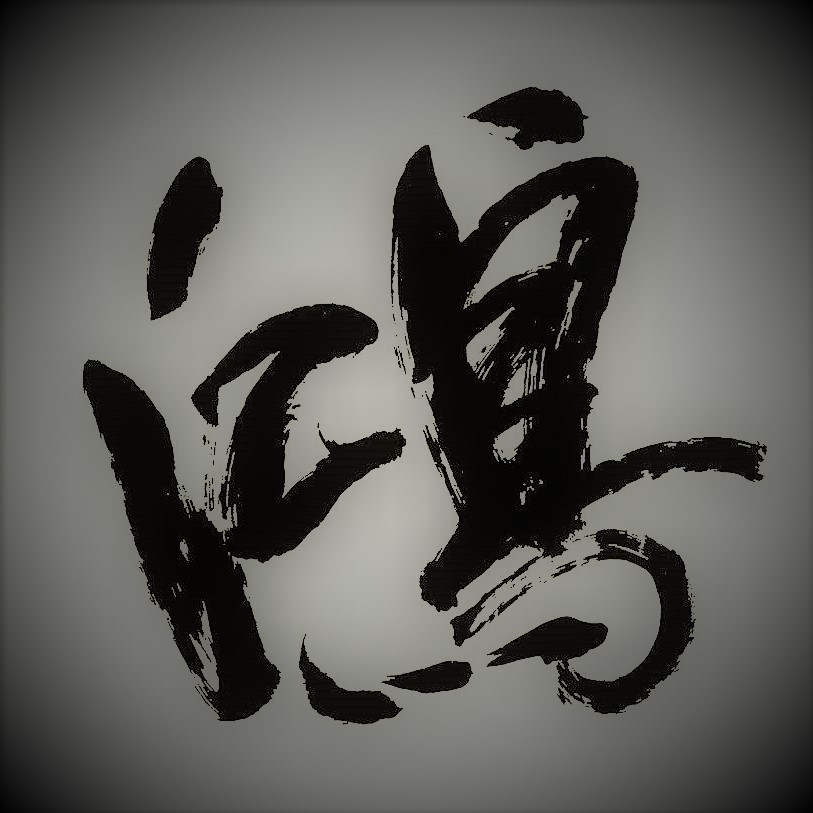# 学生时代所学的一些 C 语言知识点回顾（2）——指针耕耘实录 2019-09-10

@[toc]

# 学生时代所学的一些 C 语言知识点回顾（2）——指针

## 二 一些例子

``````# include<stdio.h>

int main(int argc,char *argv[]){
int i = 10, j, *p, *q, *x = &i;
p = &i;
q = p;
j = *&i;
printf("p = %d\n",*p);
printf("q = %d\n",*q);
printf("x = %d\n",*x);
printf("j = %d\n",j);
scanf("%d",&i);
printf("p = %d\n",*p);
printf("q = %d\n",*q);
printf("x = %d\n",*x);
printf("j = %d\n",j);
return 0;
}
/*
p = 10
q = 10
x = 10
j = 10
98
p = 98
q = 98
x = 98
j = 10
*/``````

*&i 对变量 i 使用 & 运算符产生指向指针变量的指针，对指针使用 * 运算符则可以返回到原始变量。

``````# include<stdio.h>

int main(int argc,char *argv[]){
int *p, *q, i = 989, j = 2019;
p = &i;
q = &j;
q = p;
printf("p = %d\n",*p);
printf("p : %p\n",p);
printf("j = %d\n",j);
printf("q = %d\n",*q);
printf("q : %p\n",q);
return 0;
}
/*
p = 989
p : 0x7fff51751e5c
j = 2019
q = 989
q : 0x7fff51751e5c
*/``````

``````# include<stdio.h>

int main(int argc,char *argv[]){
int *p, *q, i = 989, j = 2019;
p = &i;
q = &j;
*q = *p;
printf("p = %d\n",*p);
printf("p : %p\n",p);
printf("j = %d\n",j);
printf("q = %d\n",*q);
printf("q : %p\n",q);
return 0;
}
/*
p = 989
p : 0x7ffc00d359ac
j = 989
q = 989
q : 0x7ffc00d359a8
*/``````

``````# include<stdio.h>

int main(int argc,char *argv[]){
char i, *p;
p = &i;
scanf("%c",p);
printf("p = %c\n",*p);
printf("i = %c\n",i);
return 0;
}
/*
e
p = e
i = e
*/``````

``````# include<stdio.h>

int main(int argc,char *argv[]){
int a, b;
int *max(int *,int *);
scanf("%d%d",&a,&b);
printf("The max number is: %d\n",*max(&a,&b));
return 0;
}

int *max(int *a, int *b){
*a = *a + 5;
if (*a > *b)
return a;
else
return b;
}
/*
1 4
The max number is: 6
*/``````

``````# include<stdio.h>

int main(int argc,char *argv[]){
int a, b;
int max(int *,int *);
scanf("%d%d",&a,&b);
printf("The max number is: %d\n",max(&a,&b));
return 0;
}

int max(int *a, int *b){
*a = *a + 5;
if (*a > *b)
return *a;
else
return *b;
}
/*
1 4
The max number is: 6
*/``````

``````# include<stdio.h>

int main(int argc,char *argv[]){
int x = 2019, y=2023;
int *a = &x, *b = &y, c;
c = (*a + *b) * 2 + 20;
printf("Sum: %d\n",c);
return 0;
}
// Sum: 8104``````

``````# include<stdio.h>

int main(int argc,char *argv[]){
int p;
int example(int *);
scanf("%d",&p);
printf("%d\n",example(&p));
return 0;
}

int example(int *p){
int a = 10086;
printf("The original value is: %d\n",*p);
p = &a;
return *p;
}``````

``````# include<stdio.h>

int main(int argc,char *argv[]){
int p;
int example(int *);
scanf("%d",&p);
printf("%d\n",example(&p));
return 0;
}

int example(int *p){
printf("The original value is: %d\n",*p);
*p = 10086;
return *p;
}``````

``````# include<stdio.h>

int main(int argc,char *argv[]){
int p;
int example(const int *);
scanf("%d",&p);
printf("%d\n",example(&p));
return 0;
}

int example(const int *p){
int x = 10086;
printf("The original value is: %d\n",*p);
p = &x;
return *p;
}
/*
98
The original value is: 98
10086
*/``````

``````# include<stdio.h>

int main(int argc,char *argv[]){
int p;
int example(const int *);
scanf("%d",&p);
printf("%d\n",example(&p));
return 0;
}

int example(const int *p){
printf("The original value is: %d\n",*p);
*p = 10086;
return *p;
}``````

``````# include<stdio.h>

int main(int argc,char *argv[]){
int p;
int example(int * const);
scanf("%d",&p);
printf("%d\n",example(&p));
return 0;
}

int example(int * const p){
printf("The original value is: %d\n",*p);
*p = 10086;
// int x = 10086;
// p = &x;
return *p;
}
/*
98
The original value is: 98
10086
*/``````

``````# include<stdio.h>

int main(int argc,char *argv[]){
int p;
int example(const int * const);
scanf("%d",&p);
printf("%d\n",example(&p));
return 0;
}

int example(const int * const p){
printf("The original value is: %d\n",*p);
// *p = 10086;
// int x = 10086;
// p = &x;
return *p;
}
/*
98
The original value is: 98
98
*/``````

## 三 小结

1848人浏览
2020-03-31

1396人浏览
2018-06-14 11:26:20

1977人浏览
2018-05-29 15:28:08

1527人浏览
2018-05-28 17:38:42

779人浏览
2012-03-16 20:45:55
JAVA语言学校的危险性
728人浏览
2008-12-14 15:59:00

846人浏览
2013-09-30 14:07:00

914人浏览
2013-04-21 07:27:00

716人浏览
2013-01-13 17:55:00
0
0
407